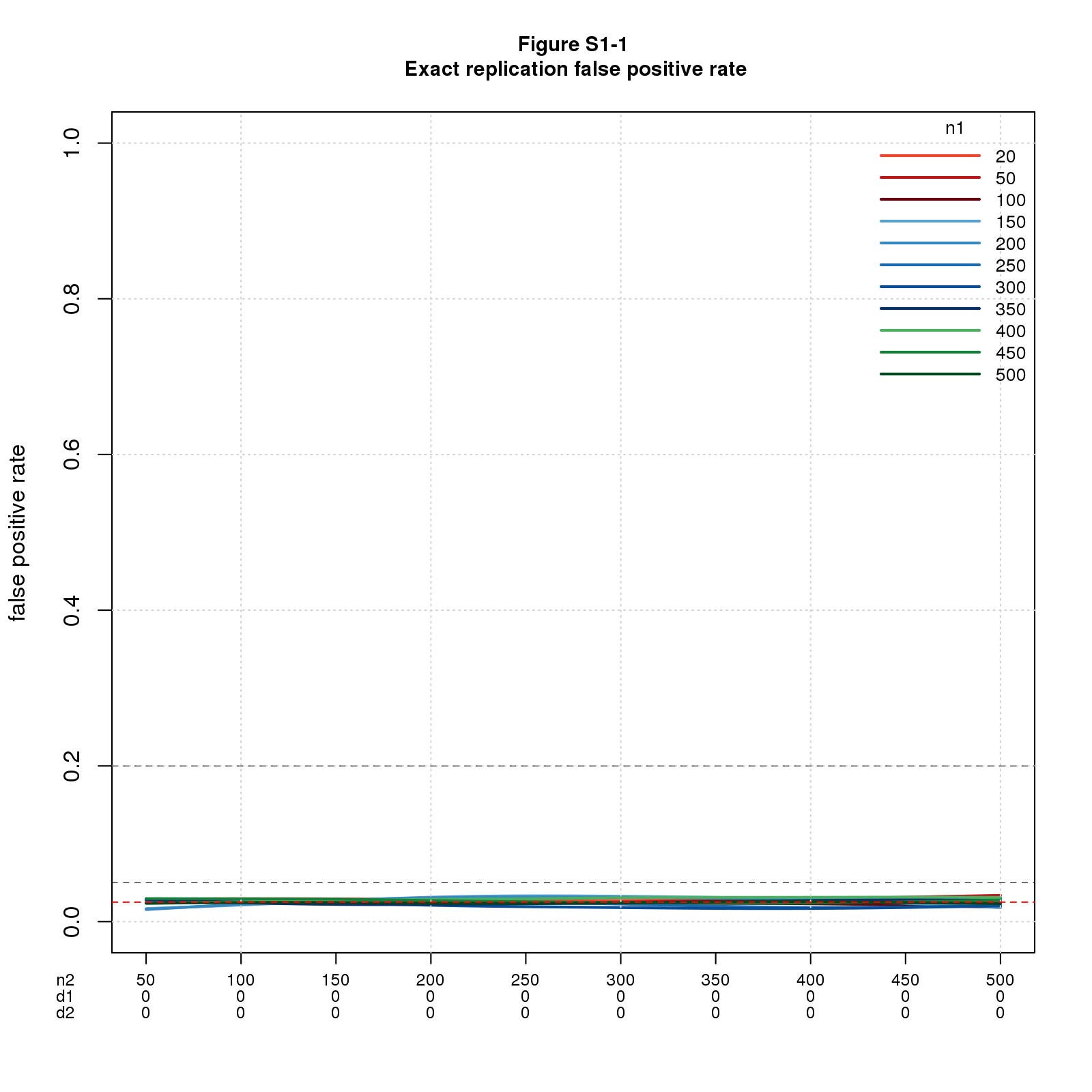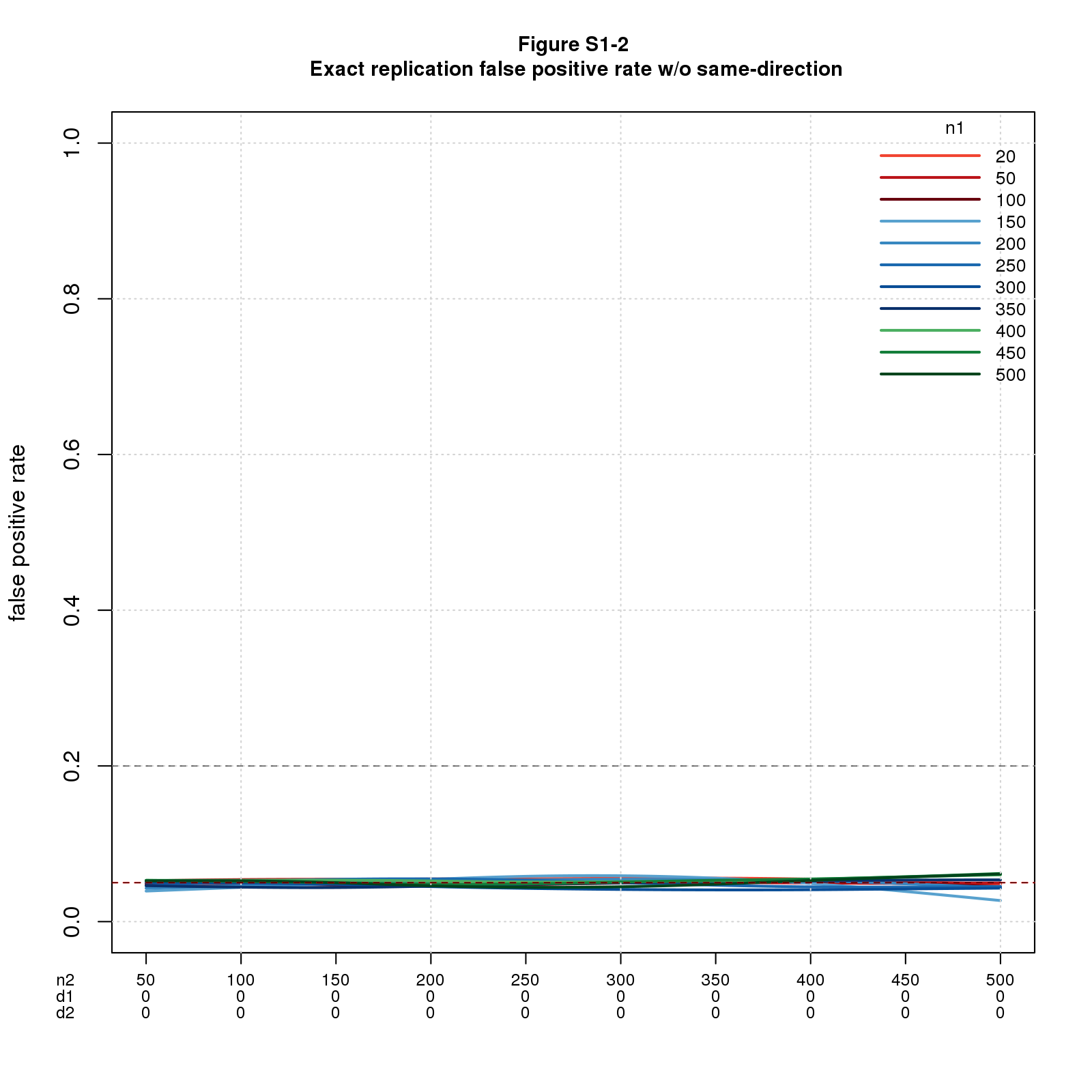## Introduction

This document contains supplementary material for the blog post Systematic Replication May Make Many Mistakes kindly posted by Bob Reed on The Replication Network. It contains results that didn’t fit in the blog post as published for reasons of space or pedagogy. It’s not terribly coherent as a standalone document. Sorry.

## Results

### Exact replications

A replication is exact if the two studies are sampling the same population; this means \(d1_{pop}=d2_{pop}\). For this ideal case, replication works as intuition predicts.

Theory tell us that FPR is the significance level divided by 2 (the factor of 2 because the effect sizes must have the same direction) and \(FNR=1-power2\), where \(power2\) is the power of the replica study. As we’ll see, the simulated FPR is dead-on, but the simulated FNR is not as good, esp. when \(n1\) and \(d\) are small. The discrepancy is due to the same-direction requirement.

#### False positive rate

For this simple case, only two parameters vary: \(n1\) and \(n2\). The effect sizes are constant: \(d1_{pop}=d2_{pop}=0\) throughout because this is the only way to get false positives with exact replications.

With only two parameters, I can show the whole picture in a single graph. Figure S1-1 shows FPR vs. \(n1\) and \(n2\), with \(n2\) on the x-axis and different colored lines for \(n1\). The lines fall on top of each other and are essentially constant illustrating that the sample sizes have no effect on the results. There’s a dashed red horizontal line at \(sig.level/2=0.025\) (just barely visible through the solid lines depicting FPR). The factor of 2 is due to requiring the effect sizes to have the same direction. Figure S1-2 confirms this explanation by replotting the results with the same-direction filter turned off.#### False negative rate

For this case, three parameters vary: \(n1\), \(n2\), and \(d=d1_{pop}=d2_{pop}\).

Figure S2-1a is like Figure 2 in the blog post with the addition of dashed lines depicting \(1-power2\) and with the legend moved down a smidge. It shows FNR for \(n1=20\) and \(n2\) varying from 50 to 500 (the same values as in Figure S1) but with \(d1_{pop}=d2_{pop}\) ranging from 0.1 to 1. Figure S2-1b is the same but with \(n1=200\).